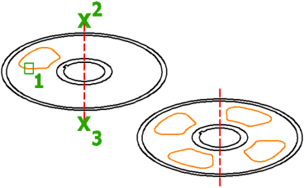# ARRAYPOLAR (Command)

Evenly distributes object copies in a circular pattern around a center point or axis of rotation.

FindThis command is equivalent to the Polar option in ARRAY. The DELOBJ system variable controls whether the source objects of the array are deleted or retained after the array is created.

The following prompts are displayed.

### Select objects

Select the objects to use in the array.

### Center point

Specifies the point around which to distribute the array items. The axis of rotation is the Z axis of the current UCS.

### Base point

Specifies a base point for the array.

Base point

Specifies a base point for positioning the objects in the array.

Key point

For associative arrays, specifies a valid constraint (or key point) on the source objects to use as the base point. If you edit the source objects of the resulting array, the base point of the array remains coincident with the key point of the source objects.

### Axis of rotation

Specifies a custom axis of rotation defined by two specified points.

### Associative

Specifies whether the arrayed objects are associative or independent.

Yes
Contains array items in a single array object, similar to a block. With an associative array, you can quickly propagate changes throughout the array by editing the properties and source objects.
No
Creates array items as independent objects. Changes to one item do not affect the other items.

### Items

Specifies the number of items in the array using a value or expression.
Note: When defining the fill angle in an expression, the (+ or -) mathematical symbol in the resultant value does not affect the direction of the array.

### Angle between

Specifies the angle between items using a value or expression.

### Fill angle

Specifies the angle between the first and last item in the array using a value or expression.

### Rows

Specifies the number of rows in the array, the distance between them, and the incremental elevation between row.

Number of rows

Sets the number of rows.

Distance between rows
Specifies the distance between each row, measured from equivalent locations on each object.
Total
Specifies the total distance between the start and end row, measured from equivalent locations on the start and end objects.
Incrementing elevation
Sets the increasing or decreasing elevation for each subsequent row.
Expression

Derives the value based on a mathematical formula or equation.

### Levels

Specifies the number and spacing of levels (for 3D arrays).

Number of levels
Specifies the number of levels in the array.
Distance between levels
Specifies the distance between the levels.
Expression

Derives a value using a mathematical formula or equation.

Total

Specifies the total distance between the first and last levels.

### Rotate items

Controls whether items are rotated as they are arrayed.

### Exit

Exits the command.